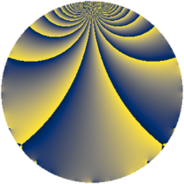# Properties

 Label 1805.2.bLevel $1805$ Weight $2$ Character orbit 1805.b Rep. character $\chi_{1805}(1084,\cdot)$ Character field $\Q$ Dimension $154$ Newform subspaces $13$ Sturm bound $380$ Trace bound $10$

# Related objects

## Defining parameters

 Level: $$N$$ $$=$$ $$1805 = 5 \cdot 19^{2}$$ Weight: $$k$$ $$=$$ $$2$$ Character orbit: $$[\chi]$$ $$=$$ 1805.b (of order $$2$$ and degree $$1$$) Character conductor: $$\operatorname{cond}(\chi)$$ $$=$$ $$5$$ Character field: $$\Q$$ Newform subspaces: $$13$$ Sturm bound: $$380$$ Trace bound: $$10$$ Distinguishing $$T_p$$: $$2$$, $$29$$

## Dimensions

The following table gives the dimensions of various subspaces of $$M_{2}(1805, [\chi])$$.

Total New Old
Modular forms 210 188 22
Cusp forms 170 154 16
Eisenstein series 40 34 6

## Trace form

 $$154 q - 138 q^{4} + 4 q^{5} - 118 q^{9} + O(q^{10})$$ $$154 q - 138 q^{4} + 4 q^{5} - 118 q^{9} - 8 q^{10} + 4 q^{11} + 12 q^{14} - 10 q^{15} + 114 q^{16} - 30 q^{20} - 20 q^{21} + 4 q^{25} - 4 q^{26} + 24 q^{29} - 24 q^{30} + 8 q^{31} - 16 q^{34} - 8 q^{35} + 106 q^{36} - 44 q^{39} + 4 q^{40} + 8 q^{41} + 28 q^{44} + 16 q^{45} - 4 q^{46} - 54 q^{49} - 4 q^{50} - 4 q^{51} - 24 q^{54} + 16 q^{55} - 52 q^{56} + 20 q^{59} - 20 q^{60} + 8 q^{61} - 34 q^{64} + 12 q^{65} + 164 q^{66} - 24 q^{69} - 16 q^{70} - 60 q^{71} - 20 q^{74} + 34 q^{75} - 16 q^{79} + 76 q^{80} - 38 q^{81} - 24 q^{84} - 24 q^{85} + 20 q^{86} + 20 q^{89} + 40 q^{90} + 32 q^{91} - 36 q^{94} + 52 q^{96} - 12 q^{99} + O(q^{100})$$

## Decomposition of $$S_{2}^{\mathrm{new}}(1805, [\chi])$$ into newform subspaces

Label Dim $A$ Field CM Traces $q$-expansion
$a_{2}$ $a_{3}$ $a_{5}$ $a_{7}$
1805.2.b.a $2$ $14.413$ $$\Q(\sqrt{-1})$$ None $$0$$ $$0$$ $$-2$$ $$0$$ $$q+iq^{2}-2q^{4}+(-1+i)q^{5}+2iq^{7}+\cdots$$
1805.2.b.b $2$ $14.413$ $$\Q(\sqrt{-1})$$ None $$0$$ $$0$$ $$-2$$ $$0$$ $$q+iq^{2}-2q^{4}+(-1-i)q^{5}-2iq^{7}+\cdots$$
1805.2.b.c $2$ $14.413$ $$\Q(\sqrt{-1})$$ None $$0$$ $$0$$ $$-2$$ $$0$$ $$q+iq^{2}+q^{4}+(-1-2i)q^{5}+2iq^{7}+\cdots$$
1805.2.b.d $2$ $14.413$ $$\Q(\sqrt{-19})$$ $$\Q(\sqrt{-19})$$ $$0$$ $$0$$ $$-1$$ $$0$$ $$q+2q^{4}+(-1+\beta )q^{5}+(-1+2\beta )q^{7}+\cdots$$
1805.2.b.e $6$ $14.413$ 6.0.16516096.1 None $$0$$ $$0$$ $$-1$$ $$0$$ $$q-\beta _{5}q^{2}+(-\beta _{1}+\beta _{3}+\beta _{4}-\beta _{5})q^{3}+\cdots$$
1805.2.b.f $6$ $14.413$ 6.0.4227136.1 None $$0$$ $$0$$ $$2$$ $$0$$ $$q+(\beta _{1}+\beta _{5})q^{2}+(-\beta _{1}-\beta _{4}-\beta _{5})q^{3}+\cdots$$
1805.2.b.g $6$ $14.413$ 6.0.4227136.1 None $$0$$ $$0$$ $$2$$ $$0$$ $$q+(\beta _{1}+\beta _{5})q^{2}+(-\beta _{1}-\beta _{4}-\beta _{5})q^{3}+\cdots$$
1805.2.b.h $8$ $14.413$ 8.0.$$\cdots$$.2 $$\Q(\sqrt{-95})$$ $$0$$ $$0$$ $$0$$ $$0$$ $$q+\beta _{1}q^{2}+\beta _{5}q^{3}+(-2+\beta _{2})q^{4}+\beta _{3}q^{5}+\cdots$$
1805.2.b.i $16$ $14.413$ $$\mathbb{Q}[x]/(x^{16} + \cdots)$$ None $$0$$ $$0$$ $$4$$ $$0$$ $$q+\beta _{1}q^{2}+\beta _{8}q^{3}+(-1+\beta _{2})q^{4}+\beta _{3}q^{5}+\cdots$$
1805.2.b.j $16$ $14.413$ $$\mathbb{Q}[x]/(x^{16} + \cdots)$$ None $$0$$ $$0$$ $$4$$ $$0$$ $$q+\beta _{1}q^{2}+\beta _{8}q^{3}+(-1+\beta _{2})q^{4}-\beta _{4}q^{5}+\cdots$$
1805.2.b.k $24$ $14.413$ None $$0$$ $$0$$ $$-3$$ $$0$$
1805.2.b.l $24$ $14.413$ None $$0$$ $$0$$ $$-3$$ $$0$$
1805.2.b.m $40$ $14.413$ None $$0$$ $$0$$ $$6$$ $$0$$

## Decomposition of $$S_{2}^{\mathrm{old}}(1805, [\chi])$$ into lower level spaces

$$S_{2}^{\mathrm{old}}(1805, [\chi]) \cong$$ $$S_{2}^{\mathrm{new}}(95, [\chi])$$$$^{\oplus 2}$$